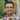# Creating a Hex Grid for HTML5 Games in Javascript

In my last post, I described how to do some of the calculations for determining the required measurements to build a hexagon. Today I’ll show you how to take that a step further and build a hex grid or game-field out of those hexagons.

Let’s start with the overview and pseudo-code for populating our grid of hexes.

#### Overview/Pseudocode:

1. Populate each row while we have vertical space on the canvas
2. After each hex (column), increment the x direction by the width of the hex + the side length until we run out of horizontal space on the canvas
3. Every other row must be offset in the x direction by the width of the hex minus the side length divided by two
4. After each row increment the y direction by 1/2 the height of the hex

#### Simple version of JavaScript code:

HT.Grid = function(/*double*/ width, /*double*/ height) {

this.Hexes = [];

var row = 0;
var y = 0.0;
while (y + HT.Hexagon.Static.HEIGHT <= height)
{
var col = 0;
var offset = 0.0;
if (row % 2 == 1)
{
offset = (HT.Hexagon.Static.WIDTH - HT.Hexagon.Static.SIDE)/2 + HT.Hexagon.Static.SIDE;
col = 1;
}

var x = offset;
while (x + HT.Hexagon.Static.WIDTH <= width)
{
//first parameter of the hexagon constructor is the hexId
//   which we'll discuss more later
var h = new HT.Hexagon(null, x, y);
this.Hexes.push(h);

col+=2;
x += HT.Hexagon.Static.WIDTH + HT.Hexagon.Static.SIDE;
}
row++;
y += HT.Hexagon.Static.HEIGHT / 2;
}

};

That is pretty straight-forward, but before we have a usable grid we may want to add a few more things. If you notice my Hexagon constructor has an “Id” member, the purpose of the Id is to give each hex a unique string identifier, this makes it easy to reference each hex, either programmatically, or for debugging. I like to give my hexes a Letter prefix based on the row plus a number based on the column, but you can do whatever works for you.

Here is my simple function for getting the hex identifiers I want:

HT.Grid.Static = {Letters:'ABCDEFGHIJKLMNOPQRSTUVWXYZ'};

HT.Grid.prototype.GetHexId = function(row, col) {
var letterIndex = row;
var letters = "";
while(letterIndex > 25)
{
letters = HT.Grid.Static.Letters[letterIndex%26] + letters;
letterIndex -= 26;
}

return HT.Grid.Static.Letters[letterIndex] + letters + (col + 1);
};

#### Hex Distance:

Finally, since it is common in games to need to figure out the distance between two hexes, we probably want a function that takes two hexagons and returns a distance. To facilitate this, we’ll need to assign some coordinates to each of our hexagons that will make it faster and easier to calculate distances when necessary. James McNeill has a very good post on his PlayTechs blog about hex grids where he describes a coordinate system for hex grids, and how to use that coordinate system to find distances between hexes.Basically, instead of a coordinate system based on the rows and columns, we have columns (shown in green), and diagonal rows (shown in red). Once we have assigned coordinates to our hexagons as shown, we can use the following equation to calculate the distances between two hexes: $\frac{|\Delta x| + |\Delta y| + |\Delta x - \Delta y|}{2}$

Here is my JavaScript function that returns the distance between two hexes in the grid:

HT.Grid.prototype.GetHexDistance = function(/*Hexagon*/ h1, /*Hexagon*/ h2) {
var deltaX = h1.PathCoOrdX - h2.PathCoOrdX;
var deltaY = h1.PathCoOrdY - h2.PathCoOrdY;
return ((Math.abs(deltaX) + Math.abs(deltaY) + Math.abs(deltaX - deltaY)) / 2);
};

Note: I’m using the PathCoOrdX and PathCoOrdY members of my Hexagons in the above function, which I populate in my full version of the Grid constructor, but I won’t go into how I do that in this post, basically the PathCoOrdX comes from the column variable, and PathCoOrdY is populated in a second pass. You can download the full Grid.js code to see the exact method I use.

#### The Fun Part:

I’ve put together a simple form and canvas, similar to what I did in my last post, so that you can see the Hex Grid in action, and play with the parameters. I’ve also added another option that allows you to switch the hex orientation that you can play with.

#### Source Files

Grid.js - The full source code for the grid class, or you can download all code for these Hexagon articles in this GitHub repo: HexagonTools.